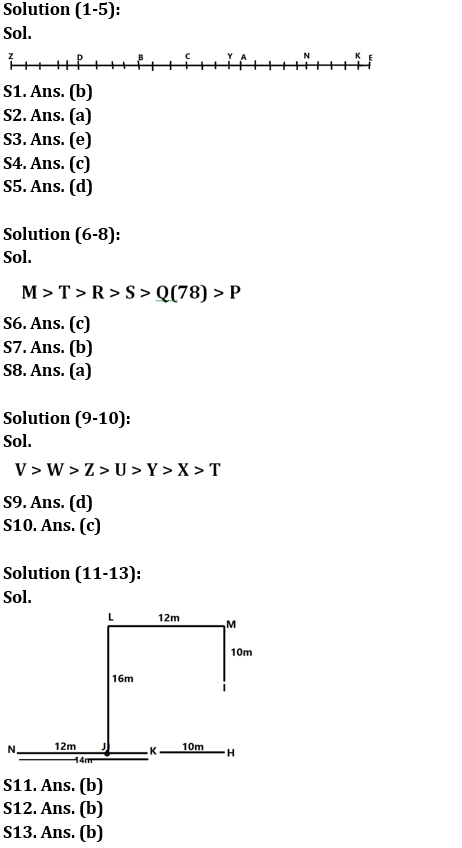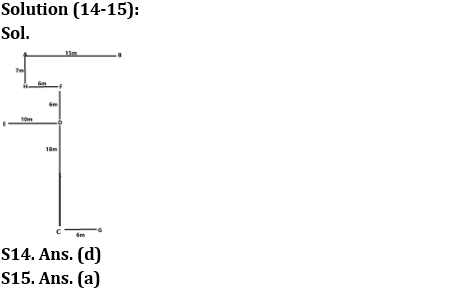Latest Banking jobs   »

# Reasoning Ability Quiz For IBPS RRB PO Clerk Prelims 2022- 20th July

Directions (1-5): Answer the questions based on the information given below.
A certain number of people sit in a straight row face in the south direction. Only six persons sit between A and B. Y sits exactly between N and B. C sits third to the left of B. D sits seventh to the right of C. Number of persons sitting between D and E is twice the number of persons sitting between D and A. D sits sixth from the right end of the row. E sits one of the extreme ends. K is an immediate neighbor of E. Only three persons sit between K and N. Z sits on one of the extreme ends.

Q1. How many persons sit in the given row?
(a) 28
(b) 27
(c) 21
(d) 17
(e) None of these

Q2. What is the position of A with respect to C?
(a) 4th to the left
(b) 4th to the right
(c) 3rd to the left
(d) 2nd to the right
(e) None of these

Q3. How many persons sit between Y and D?
(a) Three
(b) Eight
(c) Six
(d) Seven
(e) None of these

Q4. What is the position of A with respect to Z?
(a) 14th to the left
(b) 16th to the right
(c) 16th to the left
(d) 20th to the right
(e) None of these

Q5. If G sits immediate left of D and F sits immediate right of Y, then which of the following statement is true?
(a) Only five persons sit between G and F
(b) Only three persons sit between C and F
(c) Only seven persons sit between N and G
(d) Only ten persons sit between F and K
(e) None of these

Direction (6-8): Answer the questions based on the information given below.
There are six persons R, S, M, P, Q, and T get different marks in an examination. R got only fewer marks than M and T. S got marks more than Q who did not get the least marks in the examination. The second lowest person got 78 marks. M’s marks are more than T.

Q6. What may be the possible marks obtained by P?
(a) 79
(b) 80
(c) 65
(d) 85
(e) None of these

Q7. How many persons got more marks than S?
(a) Two
(b) Three
(c) Five
(d) Four
(e) One

Q8. Who scored the third lowest marks?
(a) S
(b) R
(c) T
(d) Q
(e) Can’t be determined

Direction (9-10): Answer the questions based on the information given below.
Seven batsmen T, U, V, W, X, Y & Z scored different runs in a match. Y scored more runs than two batsmen. U scored more runs than T & X but less than Z. Z scored lesser runs than W & V. W is not the highest scorer. X is not the lowest scorer.

Q9. Who is the 2nd highest scorer?
(a) Z
(b) Y
(c) X
(d) W
(e) V

Q10. How many batsmen scored less runs than U?
(a) 2
(b) 3
(c) 4
(d) 5
(e) None of the above

Direction (11-13): Answer the questions based on the information given below.
Seven hockey players H, I, J, K, L, M & N are at different positions with each other in a hockey ground. N is 14m west of K who is 10m west of H. J is east of N. The distance between H and N is twice the distance between J and N. L is 16m north of J. M is 12m east of L. M is 10m north of I.

Q11. What is the shortest distance between J and H?
(a) 18m
(b) 12m
(c) 16m
(d) 14m
(e) None of the above

Q12. In which direction is L with respect to K?
(a) North-east
(b) North-west
(c) South-east
(d) South-west
(e) None of the above

Q13. In which direction is M with respect to H?
(a) North-east
(b) North
(c) South-east
(d) South
(e) None of the above

Direction (14 – 15): Answer the questions based on the information given below.
Nine persons A, B, C, D, E, F, G, H & I are marked in a city. E is 10m west of D. H is 7m south of A which is 15m west of B. G is 6m east of C which is 18m south of D. H is 6m west of F which is 6m north of D.

Q14. In which direction is E with respect to H?
(a) North-east
(b) North-west
(c) South-east
(d) South-west
(e) None of the above

Q15. In which direction is B with respect to C?
(a) North-east
(b) North-west
(c) South-east
(d) South-west
(e) None of the above

Solutions#### Congratulations!Incorrect details? Fill the form again here

•Quantitative Aptitude Quiz For IBPS RRB ...
•Reasoning Ability Quiz For IBPS RRB PO C...
•Quantitative Aptitude Quiz For IBPS RRB ...
•Reasoning Ability Quiz For IBPS RRB PO C...
•Quantitative Aptitude Quiz For IBPS RRB ...
•Reasoning Ability Quiz For IBPS RRB PO C...